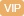| APP | 校园号 | 客服 客服热线：400-863-9889

400-863-9889（2019春•沙河口区期末）找规律填空．
1.2+a，2.4+2a，4.8+4a，
9.6+8a
9.6+8a
【考点】数列中的规律【专题】探索数的规律．

 9 4
x=
 27 16
，根据等式性质两边同乘
 4 9

 1 2
，再同以2即；
【分析】根据式性质，两边同加上
 4 5
x，得
 3 4
+
 4 5
x=
 42 25
，边同减去
 3 4
，同乘
 5 4

 3 21
x=
 6 7
×
 9 35
，即
 1 7
x=
 6 7
×
 9 35

 1 10
x=0.6，再据等式的性质两同乘即可．
【解答】解：
 7 25
×6-
 4 5
x=
 3 4

.36：x=
 1 10
 4 9

 4 5
x=
 93 100

2x-
 1 2
=.5

 3 21
x=
 6 7
×
 9 35

2-
 1 2
+
 1 2
0.5+
 1 2

x=
 3 4

 6 7
 3 21
=x：
 9 35

x÷2=1÷2
（+x）×=2×5
x=
 54 35

x=15

 3 4
+
 4 5
x-
 3 4
=
 42 25
-
 3 4

 3 4
+
 4 5
x=
 42 25

x=
 1 2

1+x.5
x+
 5 4
x=
 27 16

 4 5
 5 4
=
 93 100
×
 5 4

 9 4
 4 9
=
 27 16
×
 4 9

 1 10
x×1=0.3×10
（1+x）4÷=2×5÷4
2：1+x）=
 4 5

=3.6
【点评】此题主要考了式的性质方程的能力即式两同加或同时减去、同时乘以或同时除以个数（0除外），两边仍相．

0/0